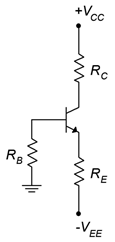# 5.7: Exercises

$$\newcommand{\vecs}{\overset { \rightharpoonup} {\mathbf{#1}} }$$ $$\newcommand{\vecd}{\overset{-\!-\!\rightharpoonup}{\vphantom{a}\smash {#1}}}$$$$\newcommand{\id}{\mathrm{id}}$$ $$\newcommand{\Span}{\mathrm{span}}$$ $$\newcommand{\kernel}{\mathrm{null}\,}$$ $$\newcommand{\range}{\mathrm{range}\,}$$ $$\newcommand{\RealPart}{\mathrm{Re}}$$ $$\newcommand{\ImaginaryPart}{\mathrm{Im}}$$ $$\newcommand{\Argument}{\mathrm{Arg}}$$ $$\newcommand{\norm}{\| #1 \|}$$ $$\newcommand{\inner}{\langle #1, #2 \rangle}$$ $$\newcommand{\Span}{\mathrm{span}}$$ $$\newcommand{\id}{\mathrm{id}}$$ $$\newcommand{\Span}{\mathrm{span}}$$ $$\newcommand{\kernel}{\mathrm{null}\,}$$ $$\newcommand{\range}{\mathrm{range}\,}$$ $$\newcommand{\RealPart}{\mathrm{Re}}$$ $$\newcommand{\ImaginaryPart}{\mathrm{Im}}$$ $$\newcommand{\Argument}{\mathrm{Arg}}$$ $$\newcommand{\norm}{\| #1 \|}$$ $$\newcommand{\inner}{\langle #1, #2 \rangle}$$ $$\newcommand{\Span}{\mathrm{span}}$$$$\newcommand{\AA}{\unicode[.8,0]{x212B}}$$

Unless otherwise specified, use $$\beta$$ = 100.

## 5.7.1: Analysis Problems

1. Plot the load line for the circuit of Figure $$\PageIndex{1}$$. $$V_{CC}$$ = 20 V, $$V_{EE}$$ = −8 V, $$R_B$$ = 7.5 k$$\Omega$$, $$R_E$$ = 10 k$$\Omega$$, $$R_C$$ = 12 k$$\Omega$$.Figure $$\PageIndex{1}$$

2. Determine the new Q point for Problem 1 if $$\beta$$ = 250.

3. Plot the load line for the circuit of Figure $$\PageIndex{2}$$. $$V_{EE}$$ = 5 V, $$V_{CC}$$ = −18 V, $$R_B$$ = 22 k$$\Omega$$, $$R_E$$ = 1.2 k$$\Omega$$, $$R_C$$ = 1.5 k$$\Omega$$.Figure $$\PageIndex{2}$$

4. Determine the new Q point for Problem 3 if $$\beta$$ = 50.

5. Plot the load line for the circuit of Figure $$\PageIndex{3}$$. $$V_{CC}$$ = 20 V, $$R_1$$ = 15 k$$\Omega$$, $$R_2$$ = 5 k$$\Omega$$, $$R_E$$ = 4.3 k$$\Omega$$, $$R_C$$ = 9.1 k$$\Omega$$.Figure $$\PageIndex{3}$$

6. Determine the new Q point for Problem 5 if $$\beta$$ = 150.

7. Plot the load line for the circuit of Figure $$\PageIndex{4}$$. $$V_{EE}$$ = 16 V, $$R_1$$ = 12 k$$\Omega$$, $$R_2$$ = 4.7 k$$\Omega$$, $$R_E$$ = 6.2 k$$\Omega$$, $$R_C$$ = 10 k$$\Omega$$.Figure $$\PageIndex{4}$$

8. Determine the new Q point for Problem 7 if $$\beta$$ = 200.

9. Plot the load line for the circuit of Figure $$\PageIndex{5}$$. $$V_{CC}$$ = 12 V, $$R_B$$ = 560 k$$\Omega$$, $$R_C$$ = 3.3 k$$\Omega$$.Figure $$\PageIndex{5}$$

10. Determine the new Q point for Problem 9 if $$\beta$$ = 75.

11. Plot the load line for the circuit of Figure $$\PageIndex{6}$$.Figure $$\PageIndex{6}$$

12. Determine the new Q point for Problem 11 if $$\beta$$ = 200.

13. Plot the load line for the circuit of Figure $$\PageIndex{7}$$. $$V_{CC}$$ = 15 V, $$R_B$$ = 470 k$$\Omega$$, $$R_E$$ = 560 $$\Omega$$, $$R_C$$ = 3.3 k$$\Omega$$.Figure $$\PageIndex{7}$$

14. Determine the new Q point for Problem 13 if $$\beta$$ = 170.

15. Plot the load line for the circuit of Figure $$\PageIndex{8}$$.

16. Determine the new Q point for Problem 15 if $$\beta$$ = 75.

17. Plot the load line for the circuit of Figure $$\PageIndex{9}$$. $$V_{EE}$$ = 18 V, $$R_B$$ = 680 k$$\Omega$$, $$R_E$$ = 270 $$\Omega$$, $$R_C$$ = 3.9 k$$\Omega$$.

18. Determine the new Q point for Problem 17 if $$\beta$$ = 200.Figure $$\PageIndex{8}$$Figure $$\PageIndex{9}$$

## 5.7.2: Design Problems

19. Determine a value for $$R_E$$ in the circuit of Figure $$\PageIndex{1}$$ to set $$I_C$$ = 2 mA. Use $$V_{CC}$$ = 20 V, $$V_{EE}$$ = −8 V, $$R_B$$ = 10 k$$\Omega$$, $$R_C$$ = 5.6 k$$\Omega$$.

20. Determine a value for $$R_C$$ in the circuit of Figure $$\PageIndex{2}$$ to set $$V_{CE}$$ = 10 V. Use $$V_{CC}$$ = −25 V, $$V_{EE}$$ = 6 V, $$R_B$$ = 15 k$$\Omega$$, $$R_E$$ = 6.8 k$$\Omega$$.

21. Determine a value for $$R_C$$ in the circuit of Figure $$\PageIndex{3}$$ to set $$V_{CE}$$ = 8 V. Use $$V_{CC}$$ = 24 V, $$R_1$$ = 22 k$$\Omega$$, $$R_2$$ = 10 k$$\Omega$$, $$R_E$$ = 5.6 k$$\Omega$$.

22. Determine new values for $$R_1$$ and $$R_2$$ in the circuit of Figure $$\PageIndex{4}$$ in order to set $$I_C$$ = 500 $$\mu$$A. $$V_{EE}$$ = 22 V, $$R_E$$ = 15 k$$\Omega$$, $$R_C$$ = 6.8 k$$\Omega$$.

## 5.7.3: Challenge Problems

23. Determine the maximum and minimum values for $$I_C$$ in Problem 1 if every resistor has a 10% tolerance.

24. Determine the maximum and minimum values for $$V_{CE}$$ in Problem 3 if every resistor has a 5% tolerance.

25. Determine a value for $$R_E$$ in the circuit of Figure $$\PageIndex{3}$$ to set $$V_{CE}$$ = 10 V. $$V_{CC}$$ = 30 V, $$R_1$$ = 12 k$$\Omega$$, $$R_2$$ = 3 k$$\Omega$$, $$R_C$$ = 8.2 k$$\Omega$$.

26. Derive Equation 5.16.

27. Determine the the power drawn from the supply for the circuit of Problem 5. 28. Using a 15 volt power supply, design a bias circuit to create a very stable Q point of 2 mA and 5 volts.

## 5.7.4: Computer Simulation Problems

29. Perform a series of DC simulations to test the Q point stability versus $$\beta$$ of the circuit of Problem 1.

30. Perform a Monte Carlo simulation to investigate the Q point stability of the circuit of Problem 5 if the emitter resistor has a 10% tolerance.

This page titled 5.7: Exercises is shared under a CC BY-NC-SA 4.0 license and was authored, remixed, and/or curated by James M. Fiore via source content that was edited to the style and standards of the LibreTexts platform; a detailed edit history is available upon request.